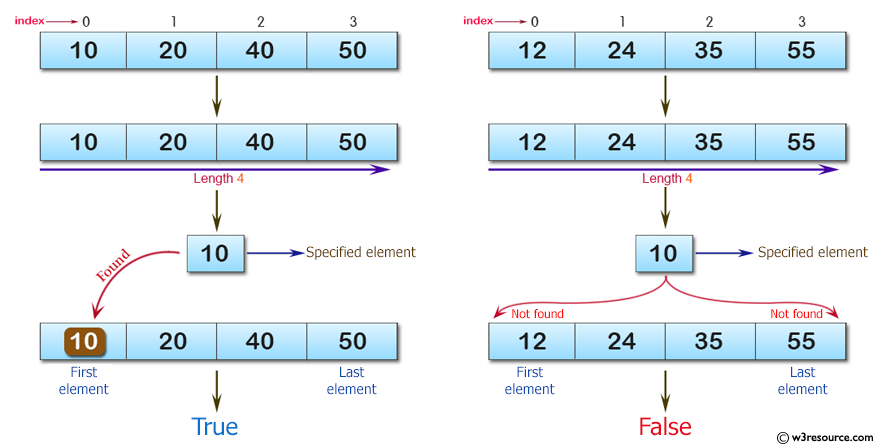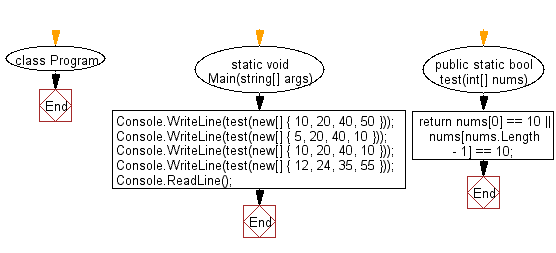﻿ C# - Check ten appears as either in first, last in an array# C# Sharp Basic Algorithm Exercises: Check a given array of integers of length 1 or more and return true if 10 appears as either first or last element in the given array

## C# Sharp Basic Algorithm: Exercise-88 with Solution

Write a C# Sharp program to check a given array of integers of length 1 or more and return true if 10 appears as either first or last element in the given array.

Pictorial Presentation:Sample Solution:-

C# Sharp Code:

``````using System;
namespace exercises
{
class Program
{
static void Main(string[] args)
{
Console.WriteLine(test(new[] { 10, 20, 40, 50 }));
Console.WriteLine(test(new[] { 5, 20, 40, 10 }));
Console.WriteLine(test(new[] { 10, 20, 40, 10 }));
Console.WriteLine(test(new[] { 12, 24, 35, 55 }));

}

public static bool test(int[] nums)
{
return nums == 10 || nums[nums.Length - 1] == 10;
}
}
}
```
```

Sample Output:

```True
True
True
False
```

Flowchart:C# Sharp Code Editor:

Improve this sample solution and post your code through Disqus

What is the difficulty level of this exercise?

Test your Programming skills with w3resource's quiz.

﻿